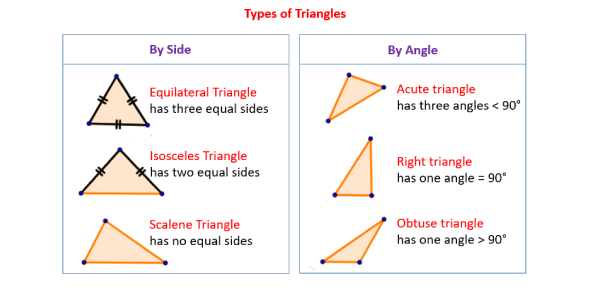# Types Of Triangles Quiz Questions

6 Questions | Attempts: 9014SettingsQuiz on the types of triangles.

• 1.
This type of triangle has one 90 degree angle.
• A.

Acute

• B.

Right

• C.

Obtuse

• 2.
A ________ triangle is one where all 3 angles measure less than 90 degrees.
• A.

Acute

• B.

Right

• C.

Obtuse

• 3.
A triangle where all sides are congruent is called
• A.

Scalene

• B.

Isosceles

• C.

Equilateral

• 4.
An isosceles triangle has
• A.

No congruent sides

• B.

2 congruent sides

• C.

3 congruent sides

• 5.
All 3 interior angles of a triangle add up to
• A.

90 degrees

• B.

180 degrees

• C.

360 degrees

• 6.
A triangle with no congruent sides and 1 angle greater than 90 degrees would be called __________ _________
• A.

Obtuse scalene

## Related TopicsBack to top
×

Wait!
Here's an interesting quiz for you.